## Articles by "RADIOACTIVITY"

Showing posts with label RADIOACTIVITY. Show all posts

The Beginning:
The history of atomic chronicle must also induce another great discovery, namely ,the Phenomenon of Radioactivity.
In 1896 the French scientist Becquerel, while investigating the nature of the mysterious X -rays discovered by Rontgen a few month earlier, found that a photographic plate wrapped in thick black paper was affected by a sample of potassium - uranyl - sulphate placed over it. In fact, any uranium compound would be effect the plate through covered by paper and kept away from light.
The oblivious conclusion that some radiations emanating from the uranium compound could penetrate through the cover and attack the photographic plate.
This penetrating radiation had its source in uranium itself and Becquerel christened this amazing behavior as Radioactivity. The properties of this radiations were very similar to those of X -rays.
1. They were highly penetrating, they effected photographic plates, they would ionize gases and would also induce the fluorescence in some substances.
2. The rays are not influenced by heat, light or chemical composition.

### Discovery of Radioactive Element:

Marie Curie found that the activity of mineral pitchblende was far greater than what was expected of its uranium content. In 1898 Pierre and Marie Curie actually isolated two new elements Polonium and Radium which were more radioactive compared to uranium, the heaviest atom known at the time.
In 1900, Debierne and Giesel discovered actinium which was also radioactive. That the radioactive effects were essentially atomic was recognized early and this helps the isolation to a considerable extent.It was immaterial how uranium and radium chemically combined. The same number of radium atoms will always have the same activity independent of the physical state or the environmental conditions. The phenomenon of radioactivity is associated with atoms which are haviour than lead or bismuth.
The phenomenon of emission of radiation as a result of spontaneous disintegration in atomic nuclei was termed as radioactivity.

### The Nature of Radiation:

The radiations emitted by naturally radioactive elements were shown to split by an electric or magnetic field into three distinct parts: Alpha(ɑ), Beta(β) and Gamma(ɣ) Rays.
• Alpha(α) Rays:
These consist a stream of positively charged particle which carry +2 charge and have mass number is 4.
These particles shown by Rutherford to be identical with, the nuclei of helium atom, that is, these are doubly charged helium ion He+2(atomic number 2, mass number 4).
When an Alpha particle ejected from within the nucleus the mother element loss two units of atomic number and four units of mass number.
 92U238 → 92U234 + 2He4
• Beta(β) Rays:
These are made up of a stream of negatively charged particles (beta particles). They have been shown to be identical with electrons from a study of their behavior in electric and magnetic fields and from the study of their e/m values (1.77 × 108 coulomb/gm).
The ejection of a beta particle (Charge -1, mass 0) results from the transformation of a neutron (mass 1, charge 0) somewhere at the surface of the nucleus into a proton (mass 1, Charge +1).
 0n1 → 1H1 + -1e0 Neutron Proton β-Rays
When a beta particle is emitted from the nucleus, the daughter element nucleus has an atomic number one unit greater than that of the mother element nucleus.
 90Th234 → 91Pa234 + -1e0
Although beta particles and electrons are identical in their electrical nature and charge/mass ratio, there is a fundamental difference between them.
Ejection of an electron from an atom converts a neutral atom into a positively charged ion but leaves the nucleus undisturbed.
Ejection of a beta particle changes the very composition of the nucleus and produces an atom of the next higher atomic number.
• Gamma Rays:
These consist of electromagnetic radiation of very short wave length (λ ∼ 0.005 - 1A). These are high energy photons.
The emission of gamma rays accompanies all nuclear reactions.
During all nuclear reactions there occurs a change in the energy of the nucleus due to emission of alpha or beta particles. The unstable, excited nucleus resulting from the emission of an alpha or beta particle gives off a photon and drops a lower and more stable energy state.
Gamma rays do not carry charge or mass, and hence emission of these rays cannot change the mass number or atomic of the mother nucleus.
• Positrons:
Since the works of the Curies and Rutherford yet another mode of nuclear transformation has been discovered. This involves the ejection of a positron +1e0 from within the nucleus.
This ejection is made possible by the conversion of a proton into neutron.
 1H1 → 0n1 + +1e0 Proton Neutron Positron
The ejection of positron lowers the atomic number one unit but leaves the mass number unchanged.
 51Sb120 → 50Sn120 + +1e0

• Neutrino:
Breaking down of a neutron into a proton and a beta particle creates a problem with the principle of conservation of angular momentum. Particles like Neutron, Proton and Electron have the spin angular momentum of ±1/2 (h/2π) each. It is thus seen that the equation:
 0n1 → 1H1 + -1

This is not balanced in so far as angular momentum is concerned. If the angular momentum of the proton and the electron are +1/2 (h/2π) they are exceed the angular momentum of the neutron.

If they oppose each other then the momentum become zero in violation of that of the neutron. Pauli therefore postulated that along with the ejected beta particle another tiny neutral particle called neutrino is also ejected. This neutrino has also spin angular momentum of ±1/2 (h/2π). The sum of angular momentum of the particles ejected {say +1/2 (h/2π) for proton, -1/2 (h/2π) for the electron and +1/2 (h/2π) for the neutrino} may now be +1/2 (h/2π) being the same as that of the neutron.
The mass of the neutrino is around 0.00002 with respect to oxygen scale.
Ejection of an electron from within the nucleus should be represented as:

 Neutron → Proton + Electron + Neutrino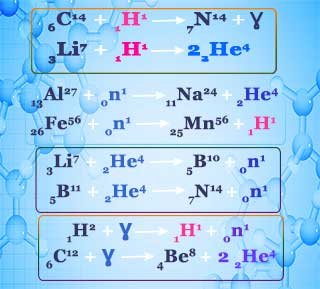Nuclear transmutation example

### Characteristics of Alpha, Beta and Gamma Rays:

 α - Ray β - Ray Ɣ - Ray 3.1×10-19 C - 1.6×10-19 C Zero +2e -1 e Zero
 6.6×10-24 gm 9.1×10-28 gm Zero 4 mH (1/1840) mH Zero
 (1.5 - 2.0) ×109 cm/sec 1010 cm/sec 3×1010cm/sec

### Difference between Nuclear and Chemical reaction:

Nuclear reactions are different from chemical reactions in many respects:
1. Chemical reactions involves some loss, gain or overlap of outer orbital electrons of the reactant atoms. Such reactions cannot alter the composition of the nuclei, so that the atomic number of the chemical reactions unchanged.
 CH4 + H2O → CO + 3H2
On the other hand Cause of Nuclear Decay involves emission of alpha particles, beta particles or positrons from inside the nucleus, which leads to change in atomic number of the nucleus.
 51Sb120 → 50Sn120 + +1e0
In some artificially induced Radioactive decay reactions, neutrons are absorbed by target nucleus producing an isotopes. Nuclear reactions therefore leads eather to the birth of another element or produce isotopes of the parent element.
2. The nuclear reactions are accompanied by energy changes which far exceed the energy changes in chemical reactions.
For example, the energy evolved in the radioactive transformation of one gram of radium is five hundred thousand times as large as the energy released when one gram of radium combine with chlorine to form RaCl2.

### Cause of radioactivity

Pairing of the Nuclear Spins:
Since radioactivity is a nuclear phenomenon it must be connected with the instability of the nucleus.
We know that the nucleus of an atom is composed of two fundamental particles, protons and neutrons. Since all elements are not radioactive, the ratio of neutron to proton of the unstable, radioactive nucleus is the factor responsible for radioactivity. Nuclear scientist studied this problems and concluded that the stability or instability is connected with the pairing of the nuclear spins.
Just as electrons spin around their own axes and just as electron- spin pairing leads to be stable chemical bonds, so also the nuclear protons and neutrons spin around their own axes, and pairing of spins of neutrons among neutrons, and pairing of spins of protons among protons leads to nuclear stability. When all the non-radioactive, stable isotopes of elements are considered it is observed that,
1. Nuclei with even number of protons and even number of neutrons are most aboundent and most stable. It is observed that even number leads to spin pairing, and odd number leads to unpaired spins.
2. Nuclei with even number of neutrons and odd number protons or odd number of neutrons and even number of protons slightly lees stable then even number of neutrons and protons.
3. By far the least stable isotopes are those which have odd numbers of protons and odd number of neutrons. The nuclear spin pairing is a maximum when odd numbers of both are present.

### Protons and Neutrons ratio:

Along with the odd or even number of protons and neutrons, the nuclear stability also influenced by the relative numbers of protons and neutrons.
The neutron/proton ratio (n/p) helps us to predict which way an unstable radioactive nucleus will decay.Ratio of protons to neutrons in stable isotopes
The above graph is obtained by plotting the number of neutrons in the nuclei of a the stable isotopes against the respective number of protons.
A study of this figure shows that the actual n/p plot of stable isotopes breaks of from hypothetical 1:1 plot around an atomic number 20, and thereafter rises rather steeply, this indicates that as the number of protons increases inside the nucleus more and more neutrons are needed to minimize the proton-proton repulsion and thereby to add to nuclear stability. Neutrons therefore serve as binding martial inside the nucleus.
The way an unstable nucleus disintegrate will be resided by its position with respect to the actual n/p plot of nuclei.
When the isotope located above this actual n/p plot it is too high an n/p ratio, and when it is located below the plot it is too low in n/p ratio. In either case the unstable nucleus should decay so as to approach the actual n/p plot. We now discuss the two cases of decay.
79Au197 is non radioactive but 88Ra226 is Radioactive-Why?
The number of neutron in gold(Au) is 118 and proton is 79.
So, n/p ratio = 118/79
=1.49

Which is lees then 1.5 thus Au-197 is stable.

But in Ra - 226 the number of Neutrons(n) = 138 and number of proton(p) = 88.
So n/p ratio = 1.57.
Thus, Ra-226 is radioactive.
• Neutron- to - Proton Ratio too High:
An isotopes with too many neutrons in the nucleus ( that is, with more neutrons than it need for stability) can attain greater nuclear stability if one of the neutrons decay to proton.
Such a disintegration leads to ejection of an electron from inside the nucleus.
 0n1 → 1H1 + -1n0(electron)
Thus beta ray emission will occur whenever the n/p ratio is higher then the n/p value expected for stability.
This is almost always the case when the mass number of the radioactive isotopes is greater then the average atomic weight of the element.
Which of the following elements are beta emitter and why?
(i) 6C12 and 6C14 (ii) 53I127 and 53I133.

1. The n/p ratio for stable carbon C-12 is 1.0(6n + 6p) but that for C - 14 is 1.3 (8n + 6p). It will predict that carbon -14 will be radioactive and will emit beta rays.
6C14 7N14 + -1e0( beta ray)
2. Similarly the n/p ratio of the stable Iodine-127 is 1.4 (74n + 53p). For Iodine-133 the n/p ratio equals 1.5 (80n + 53p) and it is again predicted to the beta emitter.
53I127 54Xe137 + -1e0(beta ray)
• Neutron- to - Proton Ratio too Low:
A nucleus deficient in neutrons will tend to attain nuclear stability by converting one of its proton to a neutron and this will be achieved either by the emission of a positron or by the capture of an electron.
 1H1 → 0n1 + +1e0(Positron)
Such Decay occurs with a radioactive isotope whose mass number is less than the average atomic weight of the element. Positron emission occurs with light isotopes of the elements of low atomic number.
 7N13 → 6C13 + +1e0 53I121 → 52Te121 + +1e0
Orbital electron capture occurs with too light isotopes ( too low n/p) of the elements of relatively high atomic number. For such elements the nucleus captures an electron from the nearest orbital ( K shell; n = 1) and thus changes one of its protons to a neutron.
 37Rb82 + -1e0 → 36Kr82 79Au194 + -1e0 → 78Pt194
Among the heaviest nuclei the total proton-proton repulsion is so large that the binding effect of neutron is not enough to lead to a stable non radioactive isotope. For such nuclei alpha particle emission is the common mode of decay.
 90Th232 → 88Ra228 + 2He4 92U235 → 90Th231 + 2He4 92U238 → 90Th234 + 2He4

### Units of radioactivity measurement:

In Practice radioactivity is expressed in terms of the number of disintegration per second. One gram of Radium undergoes about 3.7 × 10¹⁰ disintegrations per second.

The quantity of 3.7 × 10¹⁰ disintegrations per second is called curie, which is the older unit of radioactivity.
Milicurie and microcurie respectively corresponds to 3.7×10⁷ and 3.7×10⁴ disintegration per second.
Explanation:
On this basis, radioactivity of radium is 1 curie per gram. Phosphorus-32, a beta - emitter, has an activity of 50 milicuries per gram. This means that for every gram of phosphorus-32 in some material containing this species, there are 50 × 3.7 × 10⁷ disintegrations taking place per second.
1 disintegration per second is called becquerel(Bq), it is the S.I. unit of radioactivity.
Thus 1 Curie = 3.7 × 10¹⁰ Bq Another practical unit of radioactivity is Rutherford(Rd).

If a radioactive element x number disintegration per second. Express the radioactivity of this element in Curie.
3.7 × 10¹⁰ disintegrations per second = 1 curie .
Thus x number of disintegration per second = x/(3.7 × 10¹⁰) Curie.

(i) When an Alpha particle ejected from within the nucleus the mother element loss two units of atomic number and four units of mass number.
Thus, if a radioactive element with mass number M and atomic number Z ejected a alpha particle the new born element has mass number = (M - 4) and atomic number = (Z - 2).
₈₈Ra²²⁶ → ₈₈₋Rn²²²⁻⁴ He(∝)
₈₈Ra²²⁶  ₈₈Rn²²² + He(

(ii) When a beta particle is emitted from the nucleus, the daughter element nucleus has an atomic number one unit greater than that of the mother element nucleus.
Thus, if a radioactive element with mass number M and atomic number Z ejected a beta particle the new born element has mass number same and atomic number = (Z + 1).
₉₀Th²³⁴ ₉₁Pa²³⁴ + ₋₁e(β

### Disintegration Series:

We have just seen that the radioactive elements continue to undergo successive disintegration till the daughter elements becomes stable, non- radioactive isotopes of lead. The mother element along-with all the daughter elements down to the stable isotope of lead is called a radioactive disintegration series.

#### Uranium - 238 Radioactive Disintegration Series (4n + 2) Series:

Uranium - 238 decays ultimately to an isotopes of lead. The entire route involves alpha emissions in eight stages and beta emission in six stages, the overall process being,
₉₂U²³⁸ ₈₂Pb²⁰⁶ + 8₂He⁴() + 6₋₁e⁰(β
The mass number of all the above disintegration products are given by (4n +2) where n = 59 for Uranium - 238. This disintegration series is known as (4n + 2) series.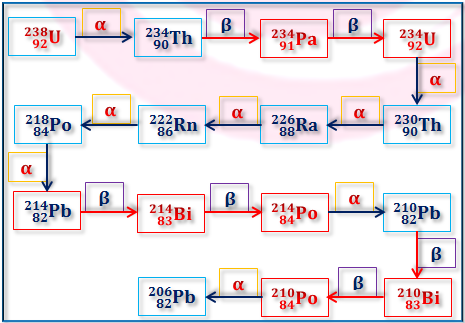Uranium - 238 Radioactive Disintegration Series or (4n+2) Series

#### Uranium - 235 Disintegration Series (4n + 3 Series):

The (4n+3) series (n=an integer) starts with Uranium - 235(n=58) and end with the stable isotope Lead - 207(n=51).
The entire route involves alpha emissions in seven stages and beta emission in four stages, the overall process being,
₉₂U²³⁵ ₈₂Pb²⁰⁷ + 7₂He⁴() + 4₋₁e⁰(β)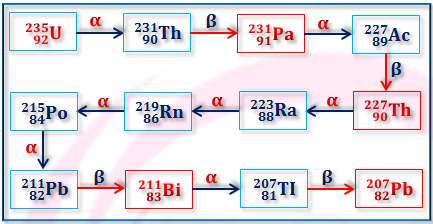Uranium - 235 Radioactive Disintegration Series or (4n+3) Series

#### Thorium- 232 Disintegration Series (4n Series):

The (4n) series (n=an integer) starts with Thorium - 232(n=58) and end with the stable isotope Lead - 208(n=52).
The entire route involves alpha emissions in six stages and beta emission in four stages, the overall process being,
₉₀Th²³² ₈₂Pb²⁰⁶ + 6₂He⁴() + 4₋₁e⁰(β)Thorium - 232 Radioactive Disintegration Series or 4n Series

### Group displacement Low:

When an alpha particle is emitted in a radioactive disintegration step, the product is displaced two places to the left in the Periodic Table but the emission of a beta particle results in a displacement of the product to one place to the right.

## Rate of Radioactive Decay:

The rate at which a radioactive sample disintegrates can be determined by counting the number of particles emitted in a given time.
Radioactive decay is one important natural phenomenon obeying the first order rate process. Rate of these reactions depends only on the single power of the conc. of the reactant. The rate can be expressed as,
 (dN/dt) = - kN
Where N is the number of the atoms of the disintegrating radio-element present at the any time, dt is the time over which the disintegration is measured and k is the radioactive decay rate constant.
 Again, k = - (dN/dt)/N
Thus, the rate constant(k) is defined as the fraction decomposing in unit time interval provided the conc. of the reactant in kept constant by adding from outside during this time interval.
The negative sign shows that N decreases with time.
Let N0 = number of the atoms present at the time t=0 and N = Number of atom present after t time interval.
Rearranging and integrating over the limits N0 and N and time, 0 and t.
 N0 t ∫ dn/N = - ∫ k dt N 0
 N0 t Therefore, ∫ d(lnN) = - ∫ k dt N 0
Thus, ln(N/N0) = -kt
or, N/N0 = e-kt
Converting to the logarithm to the base 10,
we have,  2.303 log(N/N0) = - kt
or, 2.303 log(N0/N) = kt

### Evaluation of Rate Constant(k):

Using the above equation rate constant(k) can be determined by graphical plot
of log(N0/N ) against time t.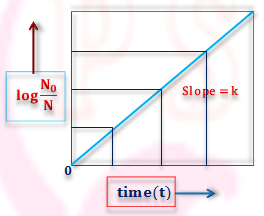Graphical Plot of log(N₀/N ) against time t

### Half Life Period of Radioactive Element:

After a certain period of time the value of (N0/N ) becomes one half, that is, half of the radioactive elements have undergone disintegration. This period is called half life of radioactive element and is a characteristic property of a radioactive element.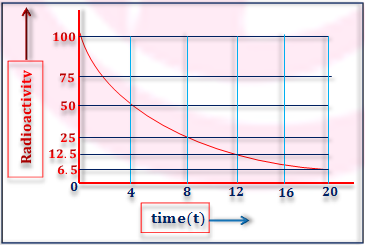Plot for Radioactive Decay
If a radioactive element is 100% radioactive and the half life period of this element 4 hour.
Thus after four hour it decompose 50% and remaining 50%. After 8 hour it decompose 75% and reaming 25% and the process is continued.
The half life is given by,
2.303 log(N0/N ) = kt
When t = t1/2N = N0/
Putting in the above equation we have,
2.303 log{N0/(N0/2)} = kt1/2
 or, t1/2 = 0.693/k  or, k = 0.693/t1/2
The above relation shows that both the half-life and radioactive decay rate constant are independent of the amount of the radio-element present at a given time.
84Po213 has t1/2 = 4.2 × 10-6 sec
whereas 83Bi209 is 3₀ × 107 years.

### Calculation of the Age Of Radioactive Elements:

(i) Age of Organic Material:
A method of determining the age of organic martial based on the accurate determination of the ratio of carbon-14 and carbon-12. carbon 14 is produced in the atmosphere by the interaction of neutron with ordinary nitrogen.
7N14 + 1n0  6C14 + 1H1
( Cosmic Reaction)
The carbon-14 ultimately goes over to carbon-14 dioxide. A steady state concentration of one 14C to 12C is reached in the atmospheric CO2 . This carbon dioxide is taken in or given out by plants and plant eating animals or human beings so they all bear this ratio.
When a plant or animals died the steady state is disturbed since there is no fresh intake of stratospheric CO2 the dead matter is out of equilibrium with the atmosphere. The 14C continues to decay so that there after a number of years only a fraction of it is left on the died matter. Therefore the ratio of the 14C/12C drops from the steady state ratio in the living matter.
6C14   7N14 + -1e0
(t1/2 = 5760 years)
By measuring this ratio and comparing it with the ratio in living plants one can estimate when the plant died.

A piece of wood was found to have ¹⁴C/¹²C ratio 0.7 times that in the living plant. Calculate the approximate period when the plant died(t₁/₂ = 5760 years).

 We know that radioactive decay constant,  k = 0.693/(t₁/₂)  = 0.693/5760 years  = 1.20 ×10⁻⁴ yr⁻¹,  and N₀/N = 1.00/0.70. ∴ 2.303 log(N₀/N) = kt or, t = (2.303 log(N₀/N)/k Putting the value, above equation, we have, t = (2.303 × 0.155)/(1.20 × 10⁻⁴)  = 2970 years

### (ii) Determination of the Age of Rock Deposits:

A knowledge of the rate of decay of certain radioactive isotopes helps to determine the age of various rock deposits. Let us consider a uranium containing rock formed many years ago.
The uranium started to decay giving rise to the uranium - 238 to lead -206 series. The half lives of the intermediate members being small compared to that of uranium -238(4.5 × 109 years), it is reasonable to assume that those uranium atoms that started decaying many many years ago must have been completely converted to the stable lead-206 during this extra long period.
The uranium-238 remaining and the lead-206 formed must together account for the uranium 238 present at zero time , that is, when the rock solidified. Thus both N0 and N are known k is known from a knowledge of the half life of uranium -238. Therefore the age of the rock can be calculated.

A sample of uranium (t₁/₂ = 4.5 × 10⁹) ore is found to contain 11.9 gm of uranium-238 and 10.3 gm of lead-206. Calculate the age of the ore.

11.9 gm of uranium-238 = 11.9/238 = 0.05 mole of uranium and 10.3 gm of lead-206 = 10.3/206 = 0.05 mole of lead -206.
Thus mole of uranium -238 present in the ore at zero time = (0.05+0.05) = 0.010 mole.
And radioactive decay constant = 0.693/(4.5 × 10⁹) = 0.154 × 10⁻⁹ yr⁻¹.
Then 2.303 log(0.10/0.05) = kt
∴ t = (2.303 log2)/(0.154 × 10⁻⁹ yr⁻¹)
= 4.5 × 10⁹ year.

### Determination of the Avogadro Number:

If 1 gm of a radioactive element contain N number of atoms.
Then, N = Avogadro Number(N0)/Mass Number(m
Therefore, kN = (k × N0)/m
Where k = Radioactive Decay Constant
= 0.693/t1/2
 Thus, kN = (0.693 × N0)/(t1/2 × m)  ∴ Avogadro Number(N0)  = (kN × t1/2 × m)/0.693
If each atom of the radio-element expels one particle then kN, the rate of decay, is also the rate at which such particles are ejected.
One gram of radium undergoes 3.7 × 1010 disintegration per second, mass number of Ra-226 = 226 and t1/2 = 1590 year, so that the Avogadro Number can be calculated by the above equation,
(3.7 × 1010 sec⁻¹ × 1590 × 365 × 24 × 60 sec × 226)/0.693
= 6.0 × 1023

### The Average Life Period(tav):

Besides half life of a radio-element another aspect of the life of a radio-element must also be known. It is now possible to determine the average life period of a radioactive atom present in an aggregate of a large number of atoms. Average life period of an atom of the radio-element tells us the average span of time after which the atom will disintegrate.
The length of time a radio-element atom can live before it disintegrates may have values from zero to infinity. This explains the gradual decay of the radio-element instead of decay of all the atoms at the same time.
The average life period may be calculated as follows-
 tav = Total Life Period/Total Number of Atoms
Let N0 atoms of a radioactive element are present in an aggregate of large no of atoms at time zero. Now in the small time interval t to (t+dt), dN atoms are found to disintegrate. Since dt is a small time period, we can take dN as the number of atoms disintegrating at the time t. So the total life time of all the dN atoms is tdN.
Again the total number of atoms N0 is composed of many such small number of atoms dN1, dN2, dN3 etc, each with its own life span t1, t2, t3 etc.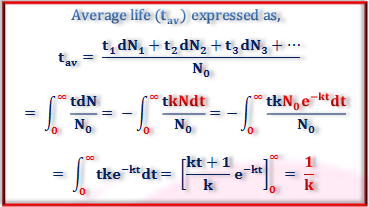Representation of Average Life Period of Radio-element
The average life of Radio-element is thus the reciprocal of its radioactive disintegration constant. This result can also be derived in a very simple alternative way. Since the radioactive atoms may be regarded as having an average life, then the product of the fractions of atoms disintegrating in unit time (that is k) and average life must be unity.
tavk = 1, So that tav = 1/k
Thus the relation between average life and half life is,
 t1/2 = 0.693/k = 0.693 tav

Name

Email *

Message *